Next: GR-Tensor Up: Tools Previous: Tools

# Penrose Diagram

Often in General Relativity one uses the Penrose diagram to describe the causal structure of the spacetime. The major feature of those diagram is to put the infinity at finite position and at the same time do so such that the null geodesics are preserved (we conserve the structure of the spacetime). One can study the global structure and the infinity.

One thing to remember is that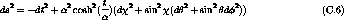is just a limit, not really there, artificial line on the Penrose diagram. It is very useful to study the causal structure but it is also dangerous because one can forget the meaning of a such diagram. Some points represents sphere and some are really just points! When using a Penrose diagram it is useful to remember these features.

Let's explain how one can obtain the Penrose diagramfor Minkowski spacetime: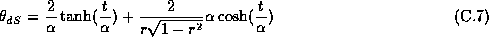The interest in this diagram is that an asymptotically flat spacetime will have the same structure as infinity and therefore the same diagram at infinity.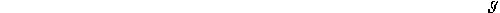Figure: Penrose diagram of Minkowski spacetime

One can remark that the null geodesics are at. We want to have t and r with a finite range and preserve this property.

We can rewrite the line element with null coordinate u and v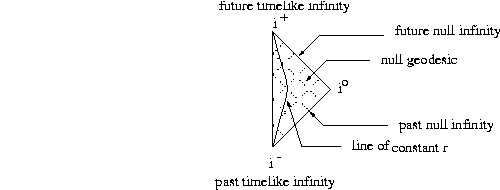and then do the transformation: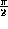with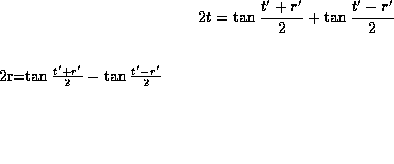,and.
With this conformal transformation  (definitionpage) we now have finite range for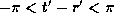andand the null geodesics are still traveling at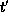.
The Penrose diagram associated with these coordinates is shown in figure.